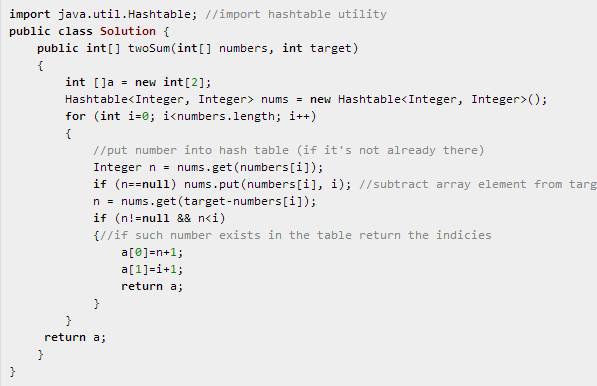# LeetCode || Two Sum

### Two Sum

Total Accepted: 16363 Total Submissions: 87273

Given an array of integers, find two numbers such that they add up to a specific target number.

The function twoSum should return indices of the two numbers such that they add up to the target, where index1 must be less than index2. Please note that your returned answers (both index1 and index2) are not zero-based.

You may assume that each input would have exactly one solution.

Input: numbers={2, 7, 11, 15}, target=9
Output: index1=1, index2=2

1、code_v1:
class Solution {
public:
vector<int> twoSum(vector<int> &numbers, int target) {
int i, j, sum;
vector<int> results;
for(i=0; (i<numbers.size()-1); i++){
//if(numbers[i]>target) continue; //这样判断不行，因为可能有负数+正数=target的情况
for(j=i+1; j<numbers.size(); j++){
//if(numbers[j]>target) continue;
sum=numbers[i]+numbers[j];
if(sum==target){
//cout<<"index1="<<(i+1)<<", index2="<<(j+1)<<endl;
results.push_back(i+1);
results.push_back(j+1);
}
}
}
return results;
}
};最终ac的代码如下：
class Solution {
public:
vector<int> twoSum(vector<int> &numbers, int target) {
int i, sum;
vector<int> results;
map<int, int> hmap;
for(i=0; i<numbers.size(); i++){
if(!hmap.count(numbers[i])){
hmap.insert(pair<int, int>(numbers[i], i));
}
if(hmap.count(target-numbers[i])){
int n=hmap[target-numbers[i]];
if(n<i){
results.push_back(n+1);
results.push_back(i+1);
//cout<<n+1<<", "<<i+1<<endl;
return results;
}

}
}
return results;
}
};

本来想用C++的hash_map，后来发现STL并没有这个容器，貌似需要自己实现代码才能用，遂用map了，map底层使用红黑树实现的，所以它的查找时间是O(logN)，略逊于hash_map，但是这不是绝对的，hash_map虽然理论上是O(N)，其实他还有计算hash值的时间消耗，也存在时间比O(logN)还大的时候（在一篇博客里看到的）。还要注意在leetcode里提交时要把cout的代码注释掉，否则可能会导致评判结果出错，本题还要注意就是map的count函数用于查找某个key是否存在，存在返回1，否则返回0；根据某个key取得其在map中的value值的方法是直接用map[key]即可。